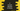# C program to convert decimal to binary## C program to convert decimal to binary:

In this post, we will learn how to convert a decimal number to binary value. The program will take the decimal number as input from the user and it will print the binary converted value.

With this program, you will learn how to take user inputs in C and how to do mathematical calculations in C.

We will learn different ways to solve this.

### How to convert a decimal value to binary:

Decimal is base 10 number system and binary is base 2 number system. We need to follow the below algorithm to convert a decimal value to binary:

• Divide the number by 2 and store the remainder in an array.
• Change the number to quotient.
• Move to the first step and keep repeating until the number is greater than 0.
• Print the array in reverse order.

Let’s take an example, Let’s convert 53 to binary.

[step] [number] [quotient for number/2] [remainder for number/2] [array]
1 53 26 1 
2 26 13 0 [1,0]
3 13 6 1 [1,0,1]
4 6 3 0 [1,0,1,0]
5 3 1 1 [1,0,1,0,1]
6 1 0 1 [1,0,1,0,1,1]
• On each step, we are changing the number to quotient of previous step.
• The remainder on each step is added to the end of the array.

Now, if we reverse the final array: 110101, it gives the binary form of 53.

Let’s see how to convert a decimal to binary in C in different ways.

## Method 1: C program to convert decimal to binary using an array:

We can use an array and put the calculated values to the array one by one. Below program uses an array to convert a decimal value to binary:

``````#include <stdio.h>

void integerToBinary(int number)
{
int i = 0, binary;

while (number > 0)
{
binary[i++] = (int)(number) % 2;
number = number / 2;
}
for (i = i - 1; i >= 0; i--)
{
printf("%d", binary[i]);
}
}

int main()
{
int num;

printf("Enter the number to convert: ");
scanf("%d", &num);

printf("\nBinary conversion:");
integerToBinary(num);

return 0;
}``````

Here,

• integerToBinary method takes one integer number and converts that to binary.
• It keeps the data in the binary array.
• The while loop gets the remainder value by using the modulo operator and puts that in binary array. It then converts the number to number/2, i.e. it assigns the quotient to the number.
• The for loop prints the binary number in a reverse format.

If you run this program, it will print output as like below:

``````Enter the number to convert: 53

Binary conversion:110101``````

## Method 2: C program to convert decimal to binary using pointer:

We can also use pointers to convert a decimal to binary in C. Let’s take a look at the program:

``````#include <stdio.h>
#include <stdlib.h>

void integerToBinary(int number)
{
int i = 0, j;
char *binary;

binary = (char *)malloc(50 * sizeof(char));

while (number > 0)
{
if ((int)(number) % 2 == 0)
{
*(binary + i) = '0';
}
else
{
*(binary + i) = '1';
}

number = number / 2;
i++;
}

for (j = i - 1; j >= 0; j--)
{
printf("%c", binary[j]);
}
}

int main()
{
int num;

printf("Enter the number to convert: ");
scanf("%d", &num);

printf("Binary conversion:");
integerToBinary(num);

return 0;
}``````

This program is almost similar to the above program. The only difference is that we are using a pointer instead of an array.

• binary is a character pointer and its memory is allocated by using malloc.
• The while loop iterates until the value of binary is more than 0.
• Inside the loop, if the remainder of dividing the number by 2 is 0, it assigns ‘0’ to that position of the pointer. Else, it assigns ‘1’ to that position.
• The for loop is printing the characters in a reverse order.

If you run this program, it will print similar output:

``````Enter the number to convert: 53
Binary conversion:110101``````

## Method 3: Return the pointer:

In the above method, we are calculating the binary and printing its value in the same method. We can also reverse the content and return it to the caller.

``````#include <stdio.h>
#include <stdlib.h>

char *integerToBinary(int number)
{
int i = 0, j, k = 0;
char *binary, *reverse;

binary = (char *)malloc(50 * sizeof(char));
reverse = (char *)malloc(50 * sizeof(char));

while (number > 0)
{
if ((int)(number) % 2 == 0)
{
*(binary + i) = '0';
}
else
{
*(binary + i) = '1';
}

number = number / 2;
i++;
}

for (j = i - 1; j >= 0; j--, k++)
{
reverse[k] = binary[j];
}

return reverse;
}

int main()
{
int num;

printf("Enter the number to convert: ");
scanf("%d", &num);

char *binary = integerToBinary(num);
printf("Binary conversion: %s", binary);

return 0;
}``````
• It puts the reverse data of binary, i.e. the required binary conversion value in reverse and returns the char pointer reverse.
• In main, we are only printing its value using %s.

If you run this program, it will print similar output.